# Math in Focus Grade 4 Chapter 6 Practice 3 Answer Key Mixed Numbers

Practice the problems of Math in Focus Grade 4 Workbook Answer Key Chapter 6 Practice 3 Mixed Numbers to score better marks in the exam.

## Math in Focus Grade 4 Chapter 6 Practice 3 Answer Key Mixed Numbers

Write a mixed number lor each model.

Example
Write a mixed number for each model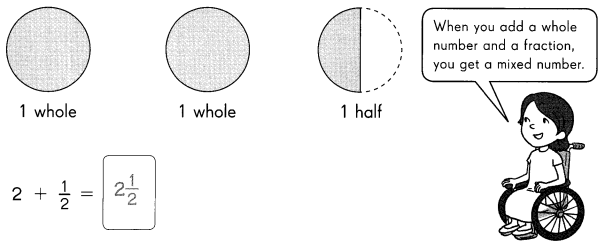Question 1.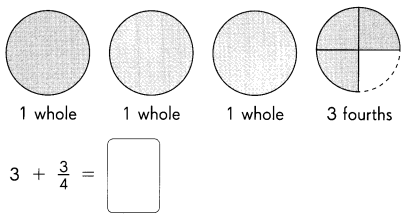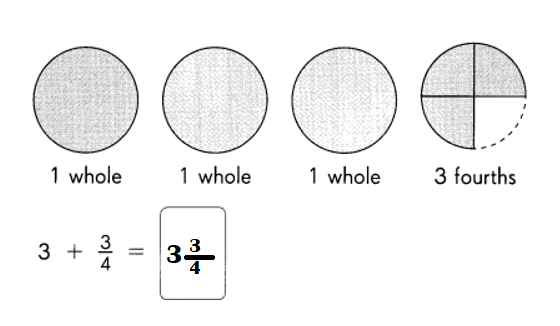Explanation:
1 + 1 + 1 + $$\frac{3}{4}$$ = 3 + $$\frac{3}{4}$$
= 3$$\frac{3}{4}$$

Question 2.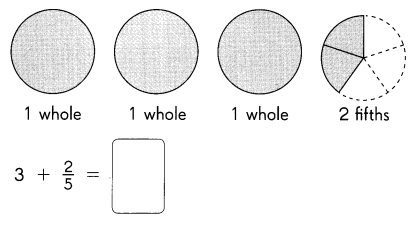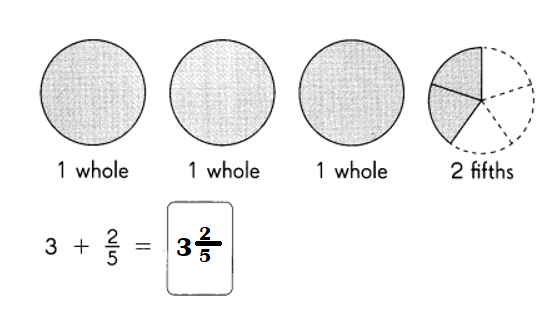Explanation:
1 + 1 + 1 + $$\frac{2}{5}$$ = 3 + $$\frac{2}{5}$$
= 3$$\frac{2}{5}$$

Write a mixed number for each model.
Question 3.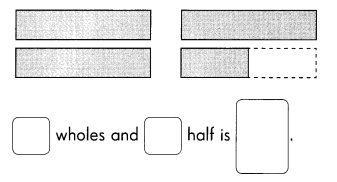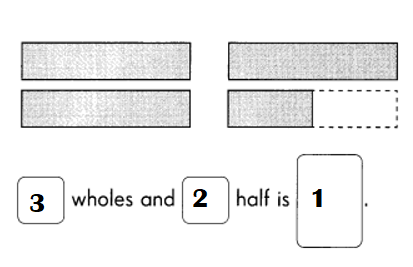Explanation:
1 + 1 + 1 + $$\frac{1}{2}$$ = 3 + $$\frac{1}{2}$$
= 3$$\frac{1}{2}$$

Question 4.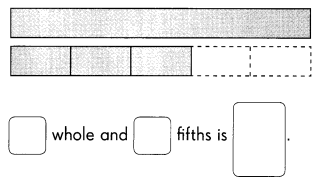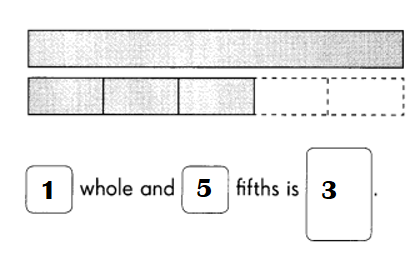Explanation:
1 + $$\frac{3}{5}$$ = 1 + $$\frac{3}{5}$$
= 1$$\frac{3}{5}$$

Question 5.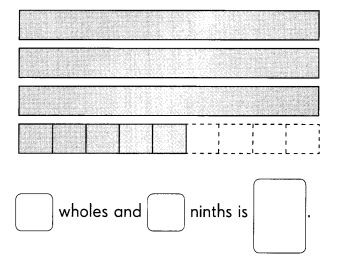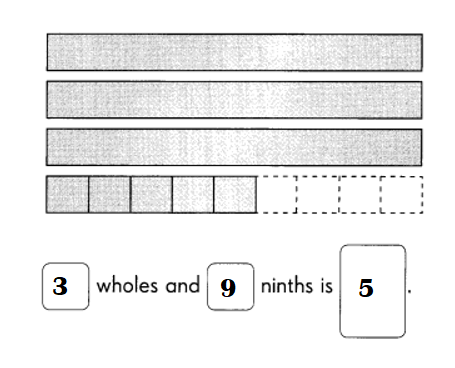Explanation:
1 + 1 + 1 + $$\frac{5}{9}$$ = 3 +$$\frac{5}{9}$$
= 3$$\frac{5}{9}$$

Check (✓) the correct model.
Question 6.
Which model shows 1$$\frac{3}{4}$$ shaded?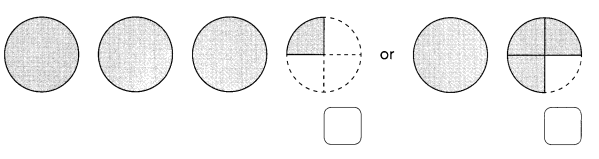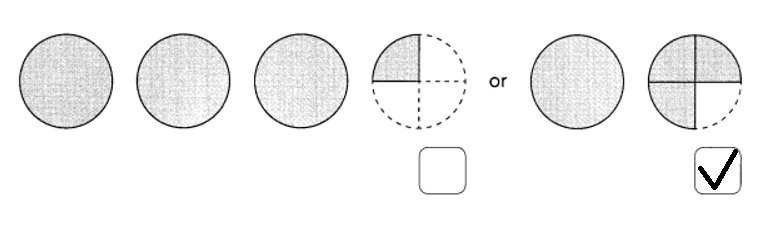Explanation:
Model 1:
1 + 1 + 1 + $$\frac{1}{4}$$ = 3 + $$\frac{1}{4}$$
= 3$$\frac{1}{4}$$

Model 2:
1 +  $$\frac{1}{4}$$ = 1 +$$\frac{1}{4}$$
= 1$$\frac{1}{4}$$

Question 7.
Which model shows 2$$\frac{3}{5}$$ shaded?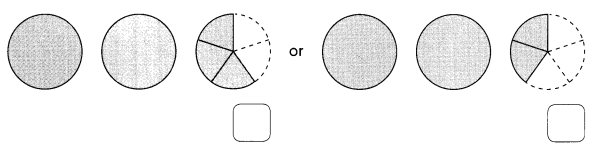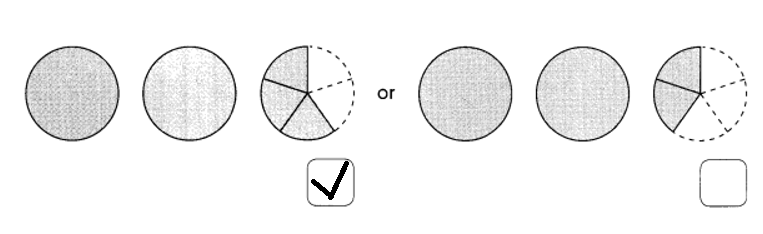Explanation:
Model 1:
1 + 1 + $$\frac{3}{5}$$ = 2 + $$\frac{3}{5}$$
= 2$$\frac{3}{5}$$
Model 2:
1 + 1 + $$\frac{2}{5}$$  = 2 + $$\frac{2}{5}$$
= 2$$\frac{2}{5}$$

Write each answer as a mixed number.
Question 8.
4 + $$\frac{1}{4}$$ = ____
4 + $$\frac{1}{4}$$ = 4$$\frac{1}{4}$$

4 + $$\frac{1}{4}$$
=4$$\frac{1}{4}$$

Question 9.
3 + $$\frac{5}{9}$$ = ____
3 + $$\frac{5}{9}$$ = 3$$\frac{5}{9}$$

Explanation:
3 + $$\frac{5}{9}$$
= 3$$\frac{5}{9}$$

Question 10.
$$\frac{5}{8}$$ + 2 = ____
$$\frac{5}{8}$$ + 2 = 2$$\frac{5}{8}$$

Explanation:
$$\frac{5}{8}$$ + 2
= 2$$\frac{5}{8}$$

Question 11.
$$\frac{3}{5}$$ + 4 = ____
$$\frac{3}{5}$$ + 4 = 4$$\frac{3}{5}$$

Explanation:
$$\frac{3}{5}$$ + 4
= 4$$\frac{3}{5}$$

Write the correct mixed number in each box.
Question 12.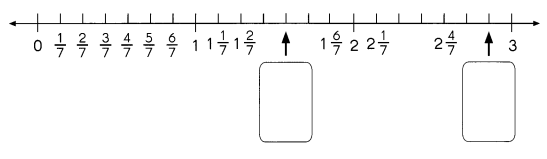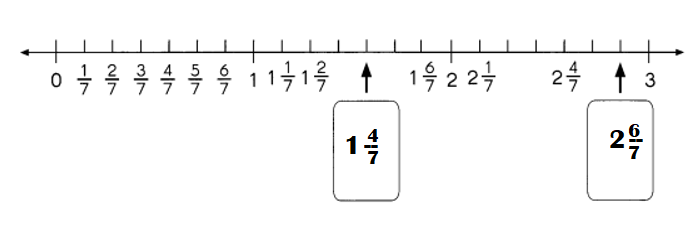Explanation:
1 + $$\frac{4}{7}$$ = 1 $$\frac{4}{7}$$
2 + $$\frac{6}{7}$$ = 2$$\frac{6}{7}$$

Write a mixed number for each item.
Question 13.
The pears have a weight of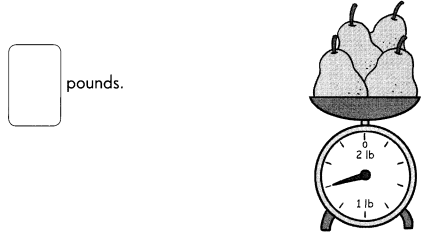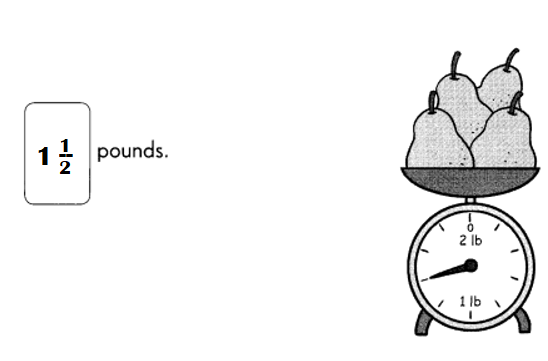Explanation:
Weight of the pears = 1 + $$\frac{2}{4}$$
= 1 $$\frac{1}{2}$$ pounds.

Question 14.
The worm started crawling from 0 centimeters.
It has crawled _____ centimeters.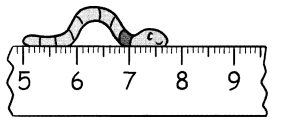It has crawled 7$$\frac{7}{1}$$ or 7.7 centimeters.

Explanation:
Number of centimeters the worm travelled = 0 + 7$$\frac{14}{2}$$
= 7$$\frac{7}{1}$$ or 7.7 centimeters.

Write each mixed number in simplest form.
Example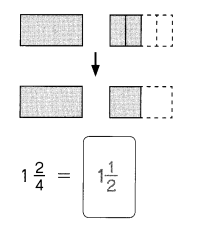Question 15.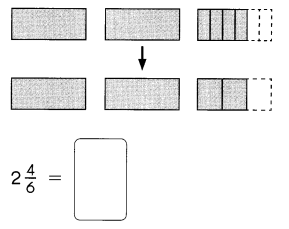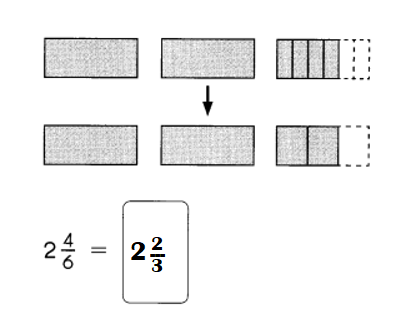Explanation:
2$$\frac{4}{6}$$ = 2$$\frac{2}{3}$$

Question 16.
3$$\frac{4}{8}$$ = ____
3$$\frac{4}{8}$$ = 3$$\frac{1}{2}$$

Explanation:
3$$\frac{4}{8}$$ = 3$$\frac{1}{2}$$

Question 17.
5$$\frac{6}{9}$$ = ____
5$$\frac{6}{9}$$ = 5$$\frac{2}{3}$$

Explanation:
5$$\frac{6}{9}$$ = 5$$\frac{2}{3}$$

Question 18.
6$$\frac{4}{12}$$ = ____
6$$\frac{4}{12}$$ = 6$$\frac{1}{3}$$

Explanation:
6$$\frac{4}{12}$$ = 6$$\frac{1}{3}$$

Question 19.
4$$\frac{3}{6}$$ = ____
4$$\frac{3}{6}$$ = 4$$\frac{1}{2}$$

Explanation:
4$$\frac{3}{6}$$ = 4$$\frac{1}{2}$$

Write each fraction and mixed number in a box to show its correct location on the number line.
Question 20.
1$$\frac{1}{2}$$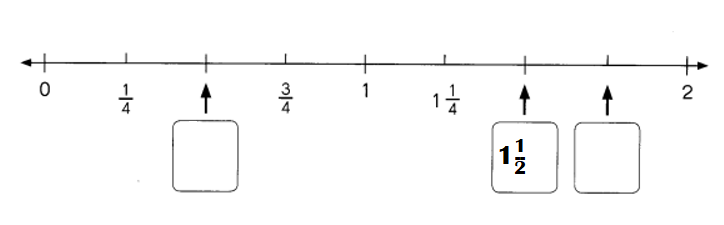Explanation:
1$$\frac{1}{2}$$ = (2 + 1) ÷ 2
= 3 ÷ 2
= 1.5.

Question 21.
$$\frac{1}{2}$$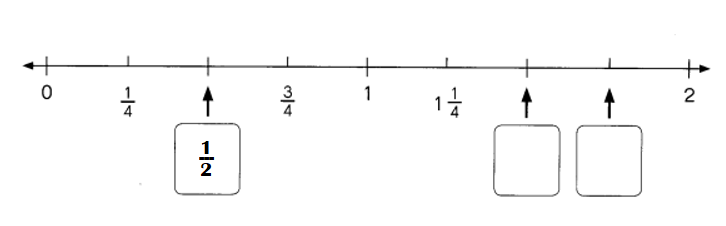Explanation:
$$\frac{1}{2}$$ = 0.5.

Question 22.
1$$\frac{3}{4}$$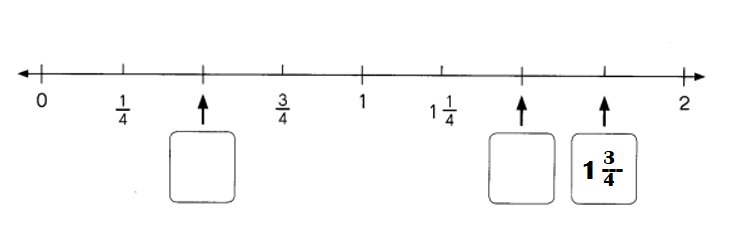Explanation:
1$$\frac{3}{4}$$ = (4 + 3) ÷ 4
= 7 ÷ 4
= 1.75.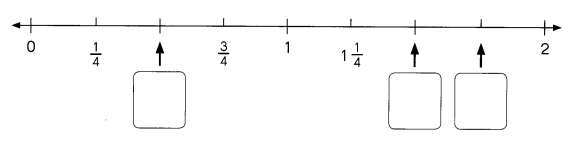Fill in the boxes with fractions or mixed numbers. Express each answer in simplest form.
Example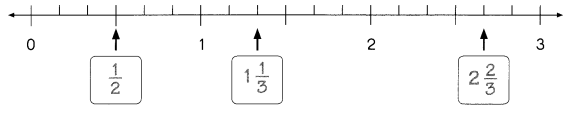Question 23.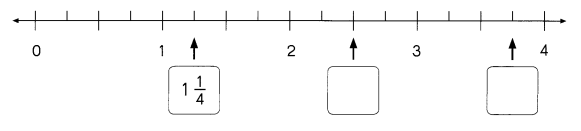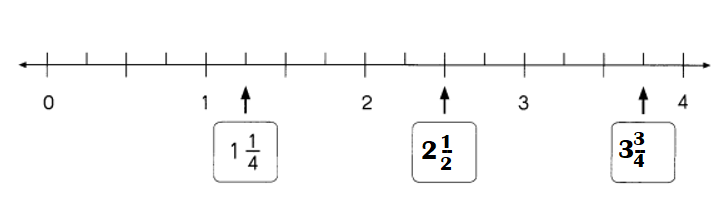Explanation:
2 + $$\frac{1}{2}$$
= 2$$\frac{2}{4}$$
= 2$$\frac{1}{2}$$

3 + $$\frac{3}{4}$$
= 3 $$\frac{3}{4}$$

Question 24.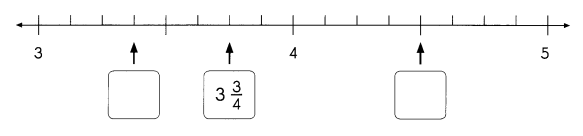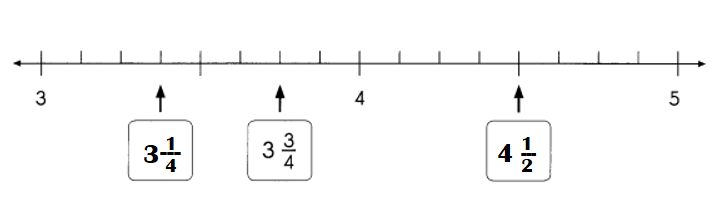Explanation:
3 +  $$\frac{1}{4}$$
= 3 $$\frac{1}{4}$$
4 + $$\frac{1}{2}$$
= 4 $$\frac{1}{2}$$

Question 25.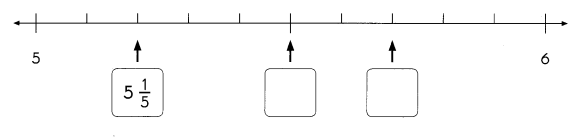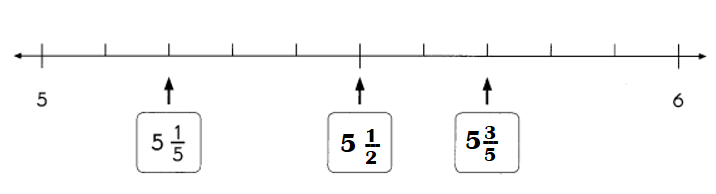5 +  $$\frac{1}{2}$$
= 5 $$\frac{1}{2}$$
5 + $$\frac{3}{5}$$
= 5 $$\frac{3}{5}$$